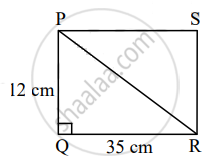# A rectangle having dimensions 35 m × 12 m, then what is the length of its diagonal? - Geometry

Sum

A rectangle having dimensions 35 m × 12 m, then what is the length of its diagonal?

#### SolutionLet □PQRS be the given rectangle.

In ∆PQR,

∠Q = 90°                     ....[Angle of a rectangle]

∴ PR2 = PQ2 + QR2    .....[Pythagoras theorem]

= 122 + 352

= 144 + 1225

= 1369

∴ PR = sqrt(1369)   ......[Taking square root of both sides]

= 37 cm

∴ The length of the diagonal of the given rectangle is 37 cm.

Concept: Converse of Pythagoras Theorem
Is there an error in this question or solution?

Share